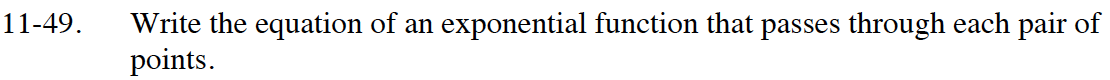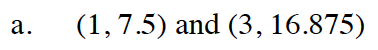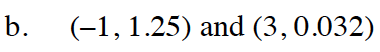Home > CC4 > Chapter 11 > Lesson 11.2.1 > Problem11-49

11-49.
1. Write the equation of an exponential function that passes through each pair of points. Homework Help ✎

2.  a. (1, 7.5) and (3, 16.875) b. (−1, 1.25) and (3, 0.032)To start, write a system of equations in the form y =abx by substituting the points in for x and y.

16.875 = ab3

7.5 = ab1

Divide the 2nd equation into the first to eliminate a.

2.25 = b2

Solve for b.

Substitute the b value you found into one of the original equations.
Solve for a.
Substitute both a and b back into the original equation with along with x and y.

y = 5(1.5)xy = 0.5(0.4)x